# 14.4: Ionization Constants of Weak Acids

Ionization Constants of Weak Acids
Acid Formula Ka at 25 °C Lewis Structure
acetic CH3CO2H 1.8 × 10−5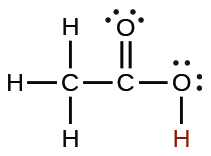arsenic H3AsO4 5.5 × 10−31.7 × 10−75.1 × 10−12
arsenous H3AsO3 5.1 × 10−10boric H3BO3 5.4 × 10−10carbonic H2CO3 4.3 × 10−75.6 × 10−11
cyanic HCNO 2 × 10−4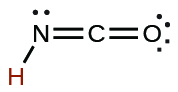formic HCO2H 1.8 × 10−4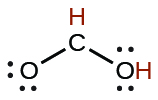hydrazoic HN3 2.5 × 10−5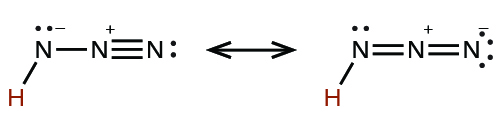hydrocyanic HCN 4.9 × 10−10
hydrofluoric HF 3.5 × 10−4
hydrogen peroxide H2O2 2.4 × 10−12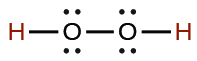hydrogen selenide H2Se 1.29 × 10−4
HSe 1 × 10−12
hydrogen sulfate ion1.2 × 10−2hydrogen sulfide H2S 8.9 × 10−8
HS 1.0 × 10−19
hydrogen telluride H2Te 2.3 × 10−3
HTe 1.6 × 10−11
hypobromous HBrO 2.8 × 10−9
hypochlorous HClO 2.9 × 10−8
nitrous HNO2 4.6 × 10−4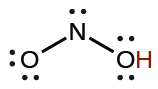oxalic H2C2O4 6.0 × 10−2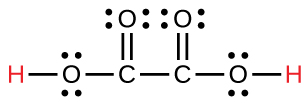6.1 × 10−5
phosphoric H3PO4 7.5 × 10−36.2 × 10−84.2 × 10−13
phosphorous H3PO3 5 × 10−2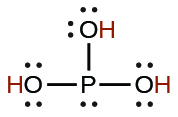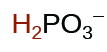2.0 × 10−7
sulfurous H2SO3 1.6 × 10−26.4 × 10−8